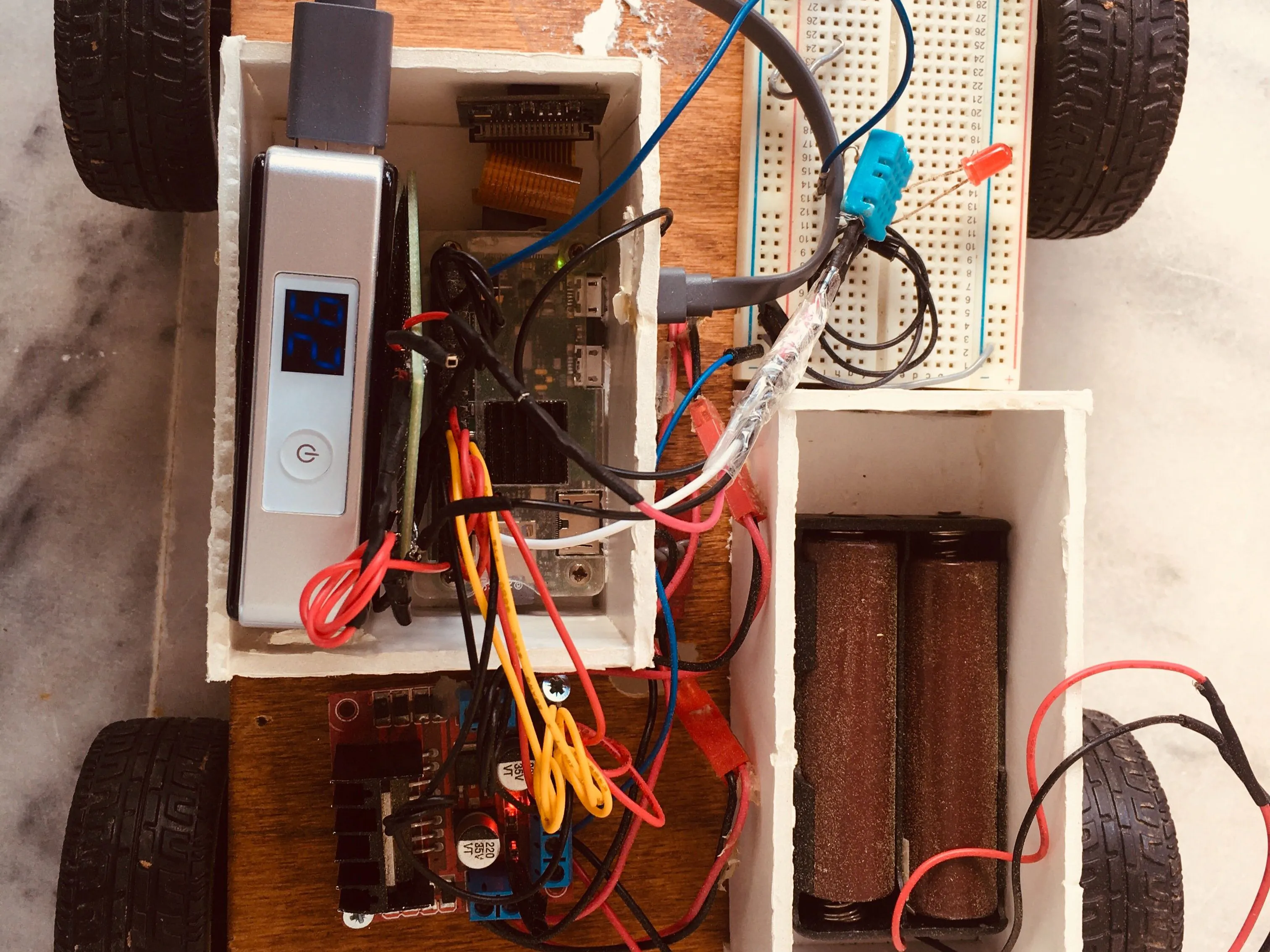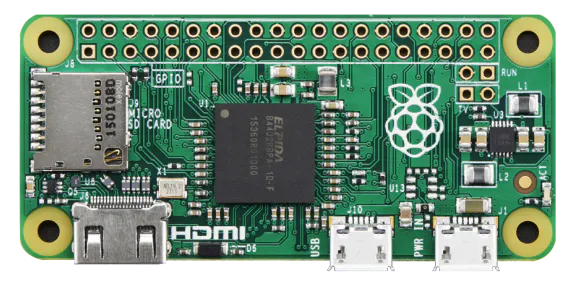Published

# Raspberypi remote controlled car

I created a raspberry pi remote-controlled car using Blynk.

BeginnerFull instructions provided5 hours642## Things used in this project

### Hardware componentsRaspberry Pi Zero
×1Battery Holder, 18650 x 2
×1
 3.7v 18650 Battery
×1
 L298N Dual Motor Driver
×1

### Software apps and online servicesRaspberry Pi RaspbianBlynk

### Hand tools and fabrication machines10 Pc. Jumper Wire Kit, 5 cm Long

## Code

### RemoteCar.py

Python
run it from raspberrypi terminal using sudo python3 remotecar.py
```import psutil
import os
import sys
import RPi.GPIO as GPIO
import time
from gpiozero import Motor,LED
from time import sleep
from gpiozero import DistanceSensor
import base64
import blynklib

in1 = 23
in2 = 24
en = 25
in3 = 17
in4 = 27
en2 = 22
start_speed=80
turn_speed=80
turn_ancle=0.3

BLYNK_AUTH = {YOUR_AUTH_CODE} #insert your Auth Token here
# base lib init
blynk = blynklib.Blynk(BLYNK_AUTH)

name = "Ragas PI"

GPIO.setmode(GPIO.BCM)
GPIO.cleanup()
GPIO.setwarnings(False)

GPIO.setup(in1,GPIO.OUT)
GPIO.setup(in2,GPIO.OUT)
GPIO.setup(in3, GPIO.OUT)
GPIO.setup(in4, GPIO.OUT)
GPIO.setup(en,GPIO.OUT)
GPIO.setup(en2,GPIO.OUT)

GPIO.output(in1,GPIO.LOW)
GPIO.output(in2,GPIO.LOW)
GPIO.output(in3,GPIO.LOW)
GPIO.output(in4,GPIO.LOW)
p=GPIO.PWM(en,1000)
p1=GPIO.PWM(en2, 1000)
p.start(start_speed)
p1.start(start_speed)

def CarForward(*args):
print("forward")
p.ChangeDutyCycle(start_speed)
p1.ChangeDutyCycle(start_speed)
GPIO.output(in1,GPIO.HIGH)
GPIO.output(in2,GPIO.LOW)
GPIO.output(in3,GPIO.HIGH)
GPIO.output(in4,GPIO.LOW)
led.on()

def CarRight(*args):
print("right")
p.ChangeDutyCycle(turn_speed)
p1.ChangeDutyCycle(turn_speed)
GPIO.output(in1,GPIO.HIGH)
GPIO.output(in2,GPIO.LOW)
GPIO.output(in3,GPIO.LOW)
GPIO.output(in4,GPIO.HIGH)
sleep(turn_ancle)
led.on()

def CarLeft(*args):
print("left")
p.ChangeDutyCycle(turn_speed)
p1.ChangeDutyCycle(turn_speed)
GPIO.output(in1,GPIO.LOW)
GPIO.output(in2,GPIO.HIGH)
GPIO.output(in3,GPIO.HIGH)
GPIO.output(in4,GPIO.LOW)
sleep(turn_ancle)
led.on()

def CarBack(*args):
print("back")
p.ChangeDutyCycle(start_speed)
p1.ChangeDutyCycle(start_speed)
GPIO.output(in1,GPIO.LOW)
GPIO.output(in2,GPIO.HIGH)
GPIO.output(in3,GPIO.LOW)
GPIO.output(in4,GPIO.HIGH)
led.on()

def CarStop(*args):
print("stop")
GPIO.output(in1,GPIO.LOW)
GPIO.output(in2,GPIO.LOW)
GPIO.output(in3, GPIO.LOW)
GPIO.output(in4,GPIO.LOW)
led.off()

def CarSpeed(*args):

global start_speed
start_speed=float(args)
print(start_speed)

def SystemEnd(*args):
GPIO.cleanup()

def stop():
CarStop()

@blynk.handle_event('write V5')
def write_virtual_pin_handler(pin, value):
val=value
if val=="1":
CarBack()
elif val=="0":
CarStop()

@blynk.handle_event('write V8')
def write_virtual_pin_handler(pin, value):
val=value
if val=="1":
CarForward()
elif val=="0":
CarStop()

@blynk.handle_event('write V7')
def write_virtual_pin_handler(pin, value):
val=value
if val=="1":
CarLeft()
elif val=="0":
CarStop()

@blynk.handle_event('write V6')
def write_virtual_pin_handler(pin, value):
val=value
if val=="1":
CarRight()
elif val=="0":
CarStop()

try:

while True:
blynk.run()

except KeyboardInterrupt:
GPIO.cleanup()
print("KeyError")

except Exception as e:
GPIO.cleanup()
print('The server is down. Try again later.'+str(e))
finally:
print("finally")
GPIO.cleanup()
```